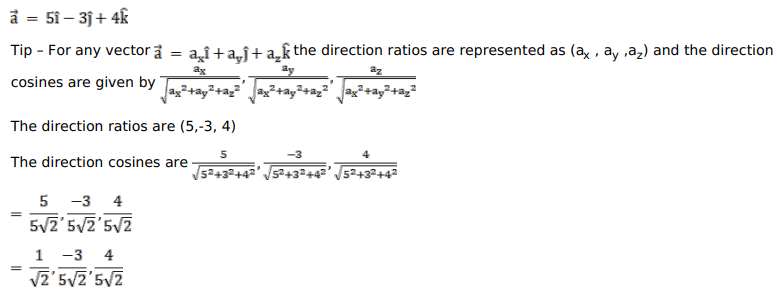# Find the direction ratios and direction cosines of the vectorQuestion:

Find the direction ratios and direction cosines of the vector $\vec{a}=(5 \hat{i}-3 \hat{j}+4 \hat{k})$.

Solution: